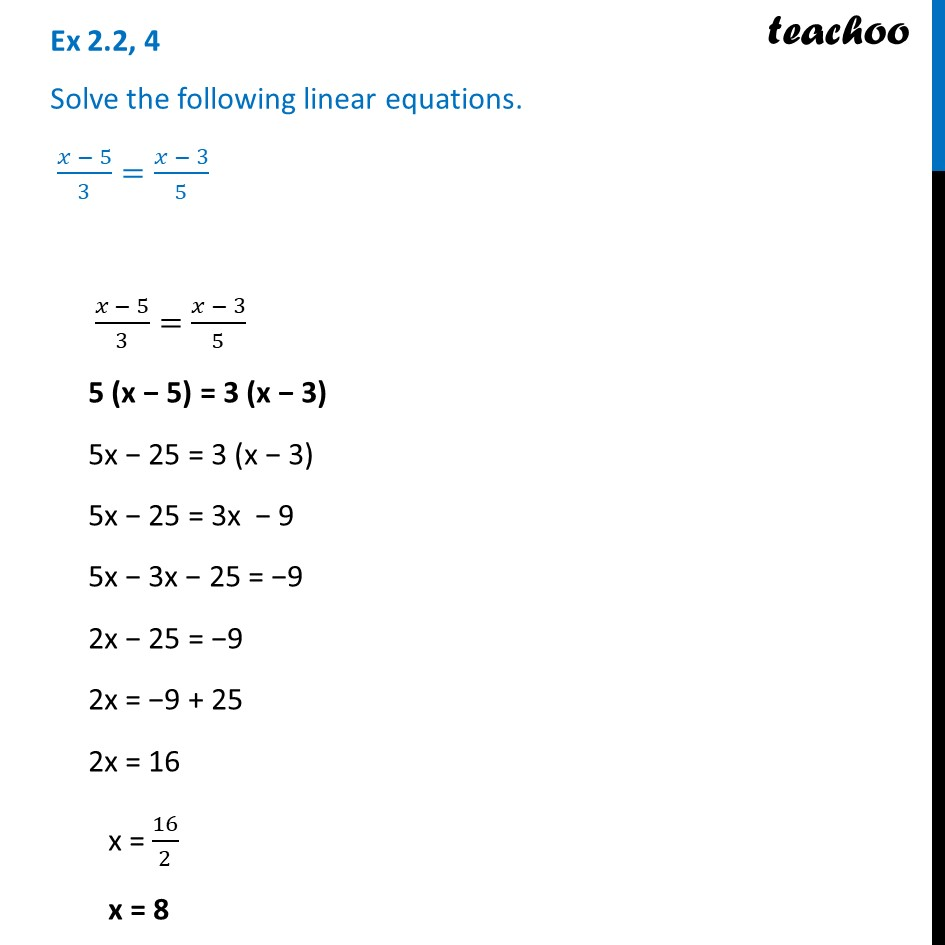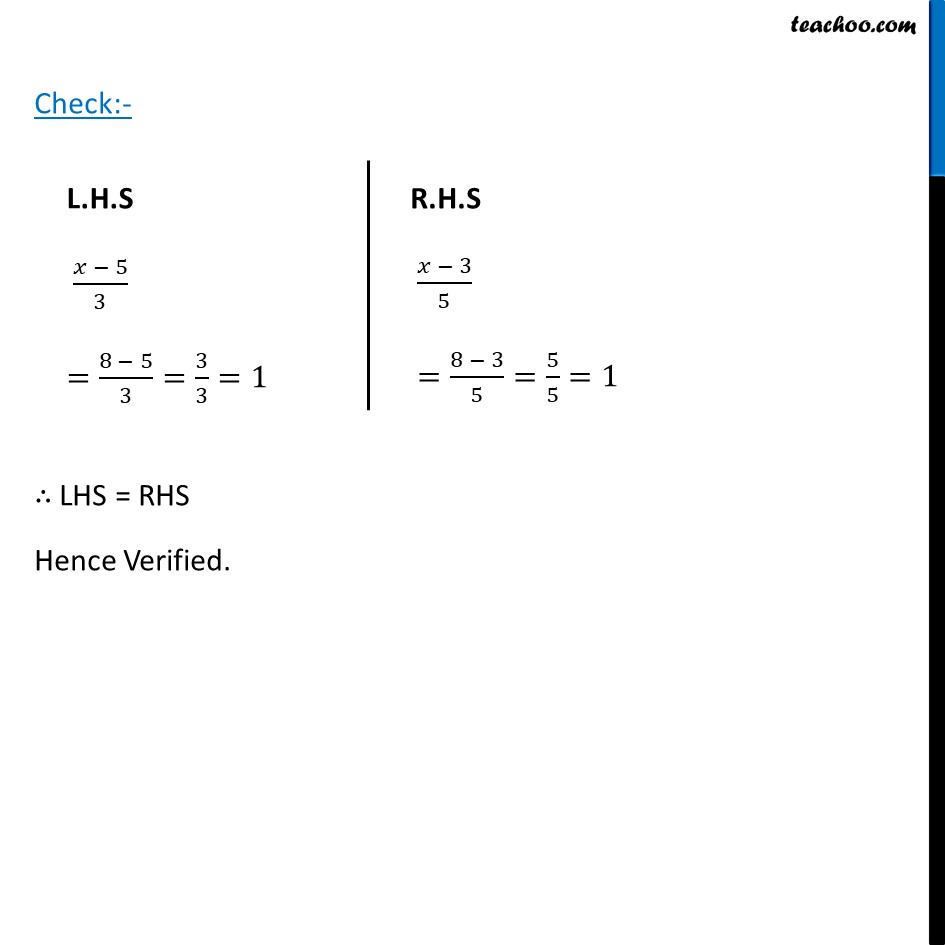Ex 2.2

Chapter 2 Class 8 Linear Equations in One Variable
Serial order wiseLearn in your speed, with individual attention - Teachoo Maths 1-on-1 Class

### Transcript

Ex 2.2, 4 Solve the following linear equations. (𝑥 − 5)/3=(𝑥 − 3)/5 (𝑥 − 5)/3=(𝑥 − 3)/5 5 (x − 5) = 3 (x − 3) 5x − 25 = 3 (x − 3) 5x − 25 = 3x − 9 5x − 3x − 25 = −9 2x − 25 = −9 2x = −9 + 25 2x = 16 x = 16/2 x = 8 Check:- ∴ LHS = RHS Hence Verified. L.H.S (𝑥 − 5)/3 =(8 − 5)/3=3/3=1 R.H.S (𝑥 − 3)/5 =(8 − 3)/5=5/5=1